CAT  >  IIFT Data Interpretation MCQ Quiz - 4

# IIFT Data Interpretation MCQ Quiz - 4

Test Description

## 18 Questions MCQ Test IIFT Mock Test Series | IIFT Data Interpretation MCQ Quiz - 4

IIFT Data Interpretation MCQ Quiz - 4 for CAT 2022 is part of IIFT Mock Test Series preparation. The IIFT Data Interpretation MCQ Quiz - 4 questions and answers have been prepared according to the CAT exam syllabus.The IIFT Data Interpretation MCQ Quiz - 4 MCQs are made for CAT 2022 Exam. Find important definitions, questions, notes, meanings, examples, exercises, MCQs and online tests for IIFT Data Interpretation MCQ Quiz - 4 below.
Solutions of IIFT Data Interpretation MCQ Quiz - 4 questions in English are available as part of our IIFT Mock Test Series for CAT & IIFT Data Interpretation MCQ Quiz - 4 solutions in Hindi for IIFT Mock Test Series course. Download more important topics, notes, lectures and mock test series for CAT Exam by signing up for free. Attempt IIFT Data Interpretation MCQ Quiz - 4 | 18 questions in 20 minutes | Mock test for CAT preparation | Free important questions MCQ to study IIFT Mock Test Series for CAT Exam | Download free PDF with solutions
 1 Crore+ students have signed up on EduRev. Have you?
IIFT Data Interpretation MCQ Quiz - 4 - Question 1

### The following bar chart gives the percentage growth in the number of households in the middle, upper middle and high income categories in the four regions for the period from 1987-88 to 1994-95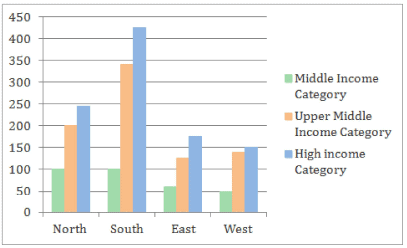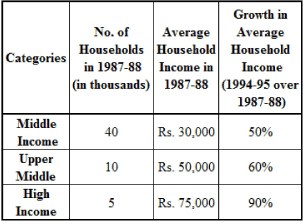Additional directions for the last two questions of this set: If the same data as the above were used, with the additional information that the numbers of households in each category were equally distributed in all the regions then Q. Which region showed the highest growth rate in the number of households in all the income categories for the period?

Detailed Solution for IIFT Data Interpretation MCQ Quiz - 4 - Question 1

question can be answered by direct observation.
For the South region, the percentage growth in each category is higher than that for every other region. Thus, the growth in number of households for all income categories is the highest for the South.
Hence, option 2.

IIFT Data Interpretation MCQ Quiz - 4 - Question 2

### The following bar chart gives the percentage growth in the number of households in the middle, upper middle and high income categories in the four regions for the period from 1987-88 to 1994-95Additional directions for the last two questions of this set: If the same data as the above were used, with the additional information that the numbers of households in each category were equally distributed in all the regions then Q. What was the total household income in the Northern Region for the upper middle class?

Detailed Solution for IIFT Data Interpretation MCQ Quiz - 4 - Question 2

Using the number of households and the average income per household for the upper middle class, the total income for the upper middle class can be found.
However, the region wise distribution of upper middle class households is not known.
Hence, the total household income in the Northern region for the upper middle class cannot be found. Hence, option 4.

IIFT Data Interpretation MCQ Quiz - 4 - Question 3

### The following bar chart gives the percentage growth in the number of households in the middle, upper middle and high income categories in the four regions for the period from 1987-88 to 1994-95Additional directions for the last two questions of this set: If the same data as the above were used, with the additional information that the numbers of households in each category were equally distributed in all the regions then Q. What is the percentage increase in the total number of households for the Northern Region (upper middle) over the given period?

Detailed Solution for IIFT Data Interpretation MCQ Quiz - 4 - Question 3

This question can be answered by direct observation.
From the bar chart, the percentage increase in the total number of households for the Northern region (upper middles class) is 200%.
Hence, option 2.

IIFT Data Interpretation MCQ Quiz - 4 - Question 4

The following bar chart gives the percentage growth in the number of households in the middle, upper middle and high income categories in the four regions for the period from 1987-88 to 1994-95Additional directions for the last two questions of this set:

If the same data as the above were used, with the additional information that the numbers of households in each category were equally distributed in all the regions then

Q.

What was the average income of the high-income group in 1987-88?

Detailed Solution for IIFT Data Interpretation MCQ Quiz - 4 - Question 4

From the table, the average income of the high income group in 1987-88 was Rs. 75,000.
Hence, option 1.

IIFT Data Interpretation MCQ Quiz - 4 - Question 5

The following bar chart gives the percentage growth in the number of households in the middle, upper middle and high income categories in the four regions for the period from 1987-88 to 1994-95Additional directions for the last two questions of this set:

If the same data as the above were used, with the additional information that the numbers of households in each category were equally distributed in all the regions then

Q.

The ratio of total income for the high income category to the upper middle class increased by how much ercent in the given period?

Detailed Solution for IIFT Data Interpretation MCQ Quiz - 4 - Question 5

In 1987-88:
The total income for the high income category households = 5000 x 75000 = Rs. 37,50,00,000 The total income for the upper middle class households = 10000 x 50000 = Rs. 50,00,00,000 Thus, the required ratio for 1987-88 = 375000000/500000000 = 3 : 4 = 0.75 It is given that the number of households in each category is equally distributed in all the regions.
Thus, there were 5000/4 = 1250 high income household in each region in 1987-88.
Similarly, there were 10000/4 = 2500 upper middle class households in each region in 1987-88.
In the year 1994-95:

The percentage growth in high income households in the four regions is 245%, 425%, 175% and 150% Thus, the number of high income households throughout the country in 1994-95 is: 1250 x (3.45 + 5.25 + 2.75 + 2.5) i.e. 1250 x 13.95 i.e 17438 households (approx) The percentage growth in upper middle class households in the four regions is 200%, 340%, 120% and 140%
Thus, the number of upper middle class households throughout the country in 1994-95 is: 2500 x (3 + 4.4 + 2.2 + 2.4) i.e. 2500 x 12 i.e 30000 households (approx) Total income for the high-income households = 17438 x 1.90 x 75000 = Rs. 2,48,49,15,000 ••• Total income for upper middle class = 30000 x 1.6 x 50000 = Rs. 2,40,00,00,000 Thus, the required ratio for 1994-95 = 2484915000/2400000000 = 1.035 Thus, the ratio has increased in absolute terms by 1.035 - 0.75 = 0.285 Hence, the required percentage increase = (0.285/0.75) x 100
= 38%
The closest option is 36%.
Hence, option 2.

IIFT Data Interpretation MCQ Quiz - 4 - Question 6

The following bar chart gives the percentage growth in the number of households in the middle, upper middle and high income categories in the four regions for the period from 1987-88 to 1994-95Additional directions for the last two questions of this set:

If the same data as the above were used, with the additional information that the numbers of households in each category were equally distributed in all the regions then

Q.

The average income for the Northern region in 1987-88, was _____ .

Detailed Solution for IIFT Data Interpretation MCQ Quiz - 4 - Question 6

The total income for the Northern region in 1987-88

= [(40000 x 30000) + (10000 x 50000) + (5000 x 75000)]/4

= (1200000000 + 500000000 + 375000000)/4

= 2075000000/4

= Rs. 51,87,50,000

The total number of household in the Norther region in 1987-88

= (40000 + 10000 + 5000)/4

= 55000/4
=13750
Thus, the average income for the Northern region in 1987-88

= 518750000/13750
= Rs. 37,727 (approx)

Hence, option 1.

IIFT Data Interpretation MCQ Quiz - 4 - Question 7

Owing to a global economic slowdown the company Testa Ltd. has decided to review its employee compensation structure in order to cut costs. In the existing structure all 100 employees are paid a Fixed Salary (FS) equal to 10000 and a Variable Salary (VS), where the Variable Salary depends upon the performance of the company and is decided as a percentage of the Fixed Salary.
Employee salary = FS + VS The company is thinking of changing it into a variable pay structure where different groups of employees are paid as shown in the table below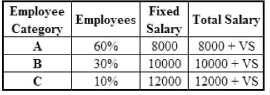Where the Variable Salary depends on the company performance and is calculated as a percentage of Fixed salary.

Q.

The company does not perform satisfactorily and decides on a variable salary = 10% of fixed salary. What is the percentage gain/loss of the company if it uses the new structure with respect to the existing  compensation structure?

Detailed Solution for IIFT Data Interpretation MCQ Quiz - 4 - Question 7

As there are 100 employees and VS = 10% of FS

In the existing structure, the total salary would be = 100 x (10000 + 10% of 10000)

= Rs. 1100000

In the proposed new structure, total salary = 60 x (8000 + 10% of 8000) + 30 x (10000 + 10% of 10000) + 10 x (12000 + 10% of 12000)

= Rs. 990000

Difference in total salary = 1100000 - 990000 = Rs. 110000

∴The company saves Rs. 110000 if it uses the new compensation structure.

∴Percentage gain = (110000/1100000) x 100 = 10%

Hence, option 3.

IIFT Data Interpretation MCQ Quiz - 4 - Question 8

Owing to a global economic slowdown the company Testa Ltd. has decided to review its employee compensation structure in order to cut costs. In the existing structure all 100 employees are paid a Fixed Salary (FS) equal to 10000 and a Variable Salary (VS), where the Variable Salary depends upon the performance of the company and is decided as a percentage of the Fixed Salary.
Employee salary = FS + VS The company is thinking of changing it into a variable pay structure where different groups of employees are paid as shown in the table belowWhere the Variable Salary depends on the company performance and is calculated as a percentage of Fixed salary.

Q.

If the variable salary is decided as 15% of the fixed salary in the existing compensation structure, what should be the percentage variable pay in the new compensation structure such that there is no difference in total employee compensation between both the structures?

Detailed Solution for IIFT Data Interpretation MCQ Quiz - 4 - Question 8

YS = 15% of FS Total employee salary in the existing compensation structure = 100 x (10000 + 15% of 10000) „ =Rs. 1150000

Let variable salary be x% o f the fixed salary in the new compensation structure

∴Total salary in the new compensation structure = 60 x (8000 + x% of 8000) + 30 x (10000 + x% of 10000) + 10 x (12000 + x% of 12000)

= Rs. (900000 + 9000x)

For there to be no difference between the two compensation structures, 1150000 = 900000 + 9000*
∴ x = (250/9) % = 27.78%

Hence, option 4.

IIFT Data Interpretation MCQ Quiz - 4 - Question 9

Owing to a global economic slowdown the company Testa Ltd. has decided to review its employee compensation structure in order to cut costs. In the existing structure all 100 employees are paid a Fixed Salary (FS) equal to 10000 and a Variable Salary (VS), where the Variable Salary depends upon the performance of the company and is decided as a percentage of the Fixed Salary.
Employee salary = FS + VS The company is thinking of changing it into a variable pay structure where different groups of employees are paid as shown in the table belowWhere the Variable Salary depends on the company performance and is calculated as a percentage of Fixed salary.

Q.

The company is not satisfied with the cost savings from the new compensation structure and decides to retrench 9 employees from employee categories of A and B such that employees retrenched from category Marks A is twice those retrenched from category B. The variable pay for the new compensation is declared to be 10%. What should be the percentage variable pay in the old compensation structure such that there is no difference between the two?

Detailed Solution for IIFT Data Interpretation MCQ Quiz - 4 - Question 9

As the company retrenches 9 employees the total employees now left are 91

Let the employees retrenched from category B be y.
Employees retrenched from category A = 2y
y + 2y = 9

∴y = 3

∴ The employees in category A, B and C are 54, 27 and 10 respectively.

Total employee compensation in the new structure = 54 x (8000 + 10% of 8000) + 27 x (10000 + 10% of 10000) + 10 x (12000 + 10% of 12000)
= Rs. 904200

Let the variable salary in the old compensation structure be z% of the fixed salary

Total employee compensation in the old structure = 91 x (10000 + z% of 10000)

= Rs. (910000 + 9100z)

For there to be no difference in the two compensation structures,

904200 = 910000 + 9100z,

which is not possible

So, the total compensation of both the structures can never be same.
Hence, option 4.

IIFT Data Interpretation MCQ Quiz - 4 - Question 10

Owing to a global economic slowdown the company Testa Ltd. has decided to review its employee compensation structure in order to cut costs. In the existing structure all 100 employees are paid a Fixed Salary (FS) equal to 10000 and a Variable Salary (VS), where the Variable Salary depends upon the performance of the company and is decided as a percentage of the Fixed Salary.
Employee salary = FS + VS The company is thinking of changing it into a variable pay structure where different groups of employees are paid as shown in the table belowWhere the Variable Salary depends on the company performance and is calculated as a percentage of Fixed salary.

Q.

If the total variable salary component of category A employees is equal to the total salary of category C employees, in the new system, then what percentage of the fixed salary is the variable salary?

Detailed Solution for IIFT Data Interpretation MCQ Quiz - 4 - Question 10

Let the variable salary be x% of the fixed salary. Then, 60 x x% of 8000 = 10 x (12000 + x% of 12000)

∴4800* = 12000 + 1200*
∴x  = 33.33%
Hence, option 1

IIFT Data Interpretation MCQ Quiz - 4 - Question 11

The two pie-charts below provide information about the background-wise batch distribution of a B-school class in 2011 and 2012. The total number of students from the Arts background increased by 25% in 2012 as compared to 2011. (Assume that the total number of students to be 300 in the year 2011)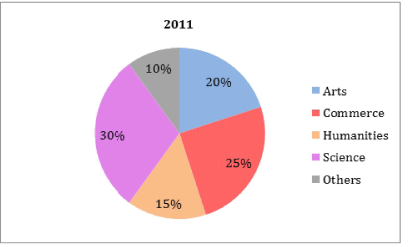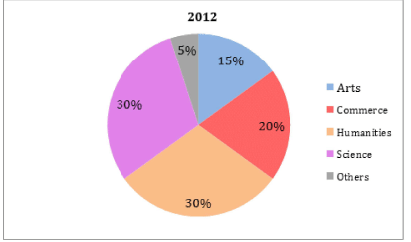Q.

How many more students were there from the Humanities background in 2012 as compared to 2011 ?

Detailed Solution for IIFT Data Interpretation MCQ Quiz - 4 - Question 11

The total number of students from the Arts background increased by 25% in 2012 as compared to 2011. Number of students from the Arts background in 2011 = 20% of 300 = 60.
Therefore, number of students from the Arts background in 2012 = 1.25 x 60 = 75 This forms 15% of the total batch size in 2012.
So, total batch size in 2012 = 75/0.15 = 500 For 300 and 500 stuents in 2011 and 2012, the number of students from each background were: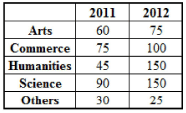Thus, there were 150 - 45 = 105 more students in the humanities background in 2012 as compared to 2011.
Hence, option 2.

IIFT Data Interpretation MCQ Quiz - 4 - Question 12

The two pie-charts below provide information about the background-wise batch distribution of a B-school class in 2011 and 2012. The total number of students from the Arts background increased by 25% in 2012 as compared to 2011. (Assume that the total number of students to be 300 in the year 2011)Q.

What was the percentage increase in the number of students from the Science background in 2012 as compared to 2011 ?

Detailed Solution for IIFT Data Interpretation MCQ Quiz - 4 - Question 12

Irrespective of the number of total students in 2011, the percentage change in the number of students from a particular background will remain the same.

Therefore, consider the solution to the previous question and assume the total number of students in 2011  to be 300.

So, the number of students from a Science background in 2011 and 2012 is 90 and 150 respectively.
Hence,

Required percentage incerase =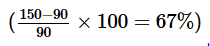Hence, option 4.

IIFT Data Interpretation MCQ Quiz - 4 - Question 13

The bar graph below shows the average electricity consumption in a house in all alternate years from 2000 to 2008. The payable amount for a particular year comprises the billed amount and 12.36% service tax. The service tax is calculated on the billed amount. The billed amount is calculated solely on the number of units consumed, and the units are billed as per various slabs (as shown in the table). For instance, if a household has consumed 420 units in a year, the first 100 units are charged at Rs. 2.23/unit, the next 300 emits are charged at Rs. 4.78/unit and the final 20 emits are charged at Rs. 6.78/unit.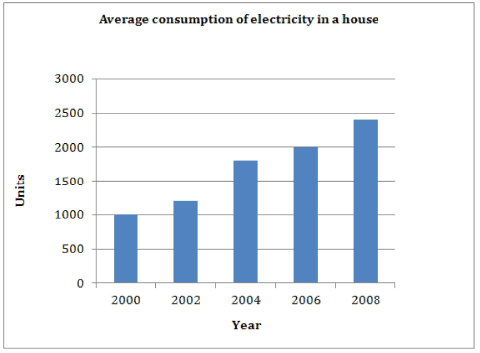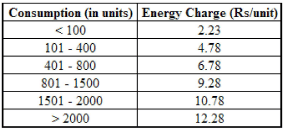Q.

What is the payable amount for this household in 2006?

Detailed Solution for IIFT Data Interpretation MCQ Quiz - 4 - Question 13

Consumption of the household in 2006 = 2000 units

∴ Billed amount = (100 x 2.23) + (300 x 4.78) + (400 x 6.78) + (700 x 9.28) + (500 x 10.78)

= 223 + 1434 + 2712 + 6496 + 5390

= Rs. 16,255

∴ Payable amount = 16255 + (12.36% of 16255)

= 1.1236 x 16255

≈ Rs. 18,264
Hence, option 2.

IIFT Data Interpretation MCQ Quiz - 4 - Question 14

The bar graph below shows the average electricity consumption in a house in all alternate years from 2000 to 2008. The payable amount for a particular year comprises the billed amount and 12.36% service tax. The service tax is calculated on the billed amount. The billed amount is calculated solely on the number of units consumed, and the units are billed as per various slabs (as shown in the table). For instance, if a household has consumed 420 units in a year, the first 100 units are charged at Rs. 2.23/unit, the next 300 emits are charged at Rs. 4.78/unit and the final 20 emits are charged at Rs. 6.78/unit.Q.

By what amount does the payable amount for 2004 exceed the payable amount for 2002?

Detailed Solution for IIFT Data Interpretation MCQ Quiz - 4 - Question 14

The number of units consumed in 2002 and 2004 is 1200 and 1800 respectively.

In 2004, the billed amount on the first 1200 units is the same as the billed amount for 2002.

So, the payable amount for the first 1200 units in 2004 is the same as the entire payable amount for 2002.

Thus, the required difference is entirely due to the additional 600 units consumed in 2004 and the service tax calculated on these 600 units.

300 of these 600 units fall in the 801-1500 slab and the remaining fall in the 1501-2000 slab.

∴Payable amount for the additional = 1.1236 x [(300 x 9.28) + (300 x 10.78)]

= 1.1236 x (2784+ 3234)

= Rs. 6761.82
Hence, option 3.

IIFT Data Interpretation MCQ Quiz - 4 - Question 15

The bar graph below shows the average electricity consumption in a house in all alternate years from 2000 to 2008. The payable amount for a particular year comprises the billed amount and 12.36% service tax. The service tax is calculated on the billed amount. The billed amount is calculated solely on the number of units consumed, and the units are billed as per various slabs (as shown in the table). For instance, if a household has consumed 420 units in a year, the first 100 units are charged at Rs. 2.23/unit, the next 300 emits are charged at Rs. 4.78/unit and the final 20 emits are charged at Rs. 6.78/unit.Q.

How much service tax has the household paid in all over the given period?

Detailed Solution for IIFT Data Interpretation MCQ Quiz - 4 - Question 15

Since the service tax rate remains constant over the given period, the service tax can be calculated directly on the total billed amount instead of calculating the service tax for each billed amount.

Consider the solution to the first question. The billed amount for 2006 is Rs. 16,255

The billed amount for the other years is:

2000: (100 x 2.23) + (300 x 4.78) + (400 x 6.78) + (200 x 9.28) = 223 + 1434 + 2712 + 1856 = Rs. 6,225

2002: (100 x 2.23) + (300 x 4.78) + (400 x 6.78) + (400 x 9.28) = 223 + 1434 + 2712 + 3712 = Rs. 8,081
9/12

2004: (100 x 2.23) + (300 x 4.78) + (400 x 6.78) + (700 x 9.28) + (300 x 10.78) = 223 + 1434 + 2712 + 6496 + 3234 = Rs. 14,099

2008: (100 x 2.23) + (300 x 4.78) + (400 x 6.78) + (700 x 9.28) + (500 x 10.78) + (400 x 12.28) = 223 + 1434 + 2712 + 6496 + 5390 + 4912 = Rs. 21,167

∴ Total billed amount = 6225 + 8081 + 14099 + 16255 + 21167 = Rs. 65,827

∴  Total service tax = 12.36% of 65827

≈ Rs. 8,136

Hence, option 3

IIFT Data Interpretation MCQ Quiz - 4 - Question 16

The table below shows the number of male and female voters in eight constituencies of a state from 2012 to 2014.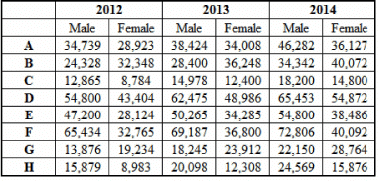Q.

In how many constituencies is the compound annual growth rate (CAGR) of male voters less than that of the female voters?

Detailed Solution for IIFT Data Interpretation MCQ Quiz - 4 - Question 16

Because, the period is only 2 years, calculate the CAGR for male and female voters using the formula: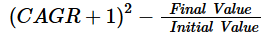Now, in a particular constituency, if the ratio on the RHS is higher for males, the males will have a higher CAGR in that constituency.

Hence, calculate only the ratio on the RHS, as shown below.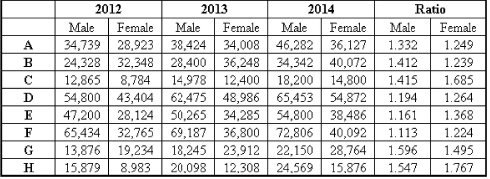Thus, the CAGR for males is less than that for females in five constituencies i.e. C, D, E, F and H.

Hence, option 3.

IIFT Data Interpretation MCQ Quiz - 4 - Question 17

The table below shows the number of male and female voters in eight constituencies of a state from 2012 to 2014.Q.

Which constituency witnessed the highest percentage growth in the total number of voters, over the given period?

Detailed Solution for IIFT Data Interpretation MCQ Quiz - 4 - Question 17

Calculate the total number of voters in 2012 and 2014, and their corresponding percentage growth as shown below: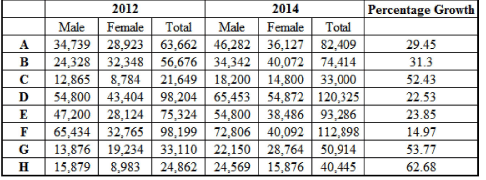Thus, the highest percentage growth is in constituency H.

Hence, option 4.

IIFT Data Interpretation MCQ Quiz - 4 - Question 18

The table below shows the number of male and female voters in eight constituencies of a state from 2012 to 2014.Q.

In which constituency is the CAGR of total voters the least over the given period?

Detailed Solution for IIFT Data Interpretation MCQ Quiz - 4 - Question 18

Consider the solution to the earlier questions.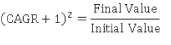Now, the final value and initial value of total voters has been found earlier, as shown below.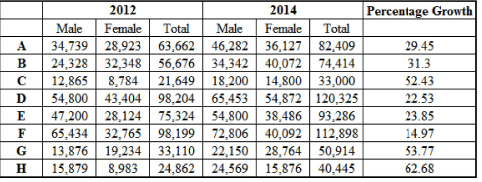As explained earlier, the constituency with the least ratio of final to initial voters will have the least CAGR.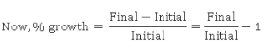Thus, the least constituency with the least percentage growth will have the least ratio, and hence, the least CAGR.
This constituency is F.

Hence, option 1.

## IIFT Mock Test Series

1 docs|46 tests
 Use Code STAYHOME200 and get INR 200 additional OFF Use Coupon Code
Information about IIFT Data Interpretation MCQ Quiz - 4 Page
In this test you can find the Exam questions for IIFT Data Interpretation MCQ Quiz - 4 solved & explained in the simplest way possible. Besides giving Questions and answers for IIFT Data Interpretation MCQ Quiz - 4, EduRev gives you an ample number of Online tests for practice

## IIFT Mock Test Series

1 docs|46 tests

### How to Prepare for CAT

Read our guide to prepare for CAT which is created by Toppers & the best Teachers